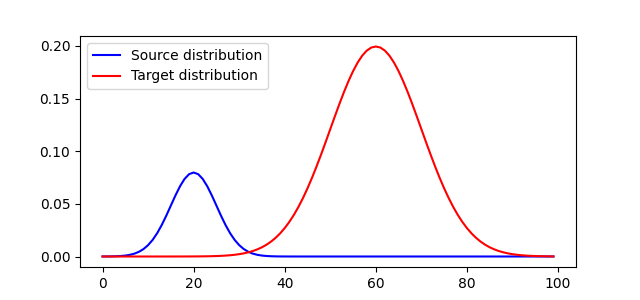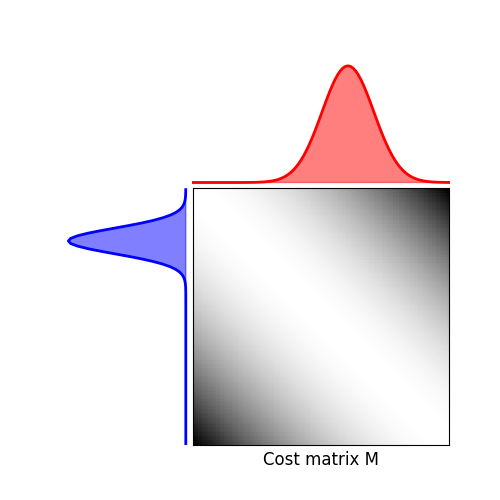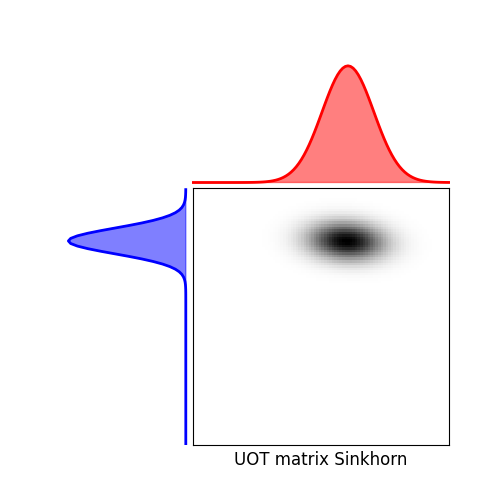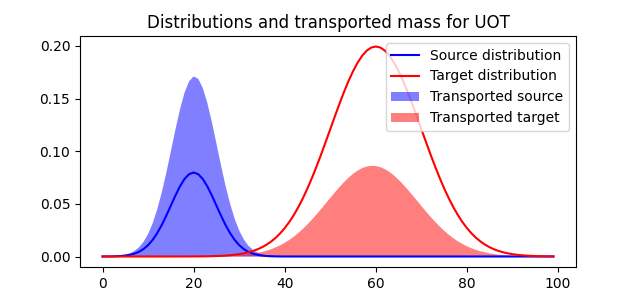# 1D Unbalanced optimal transport

This example illustrates the computation of Unbalanced Optimal transport using a Kullback-Leibler relaxation.

```# Author: Hicham Janati <hicham.janati@inria.fr>
#

# sphinx_gallery_thumbnail_number = 4

import numpy as np
import matplotlib.pylab as pl
import ot
import ot.plot
from ot.datasets import make_1D_gauss as gauss
```

## Generate data

```n = 100  # nb bins

# bin positions
x = np.arange(n, dtype=np.float64)

# Gaussian distributions
a = gauss(n, m=20, s=5)  # m= mean, s= std
b = gauss(n, m=60, s=10)

# make distributions unbalanced
b *= 5.

# loss matrix
M = ot.dist(x.reshape((n, 1)), x.reshape((n, 1)))
M /= M.max()
```

## Plot distributions and loss matrix

```pl.figure(1, figsize=(6.4, 3))
pl.plot(x, a, 'b', label='Source distribution')
pl.plot(x, b, 'r', label='Target distribution')
pl.legend()

# plot distributions and loss matrix

pl.figure(2, figsize=(5, 5))
ot.plot.plot1D_mat(a, b, M, 'Cost matrix M')
```
••## Solve Unbalanced Sinkhorn

```# Sinkhorn

epsilon = 0.1  # entropy parameter
alpha = 1.  # Unbalanced KL relaxation parameter
Gs = ot.unbalanced.sinkhorn_unbalanced(a, b, M, epsilon, alpha, verbose=True)

pl.figure(3, figsize=(5, 5))
ot.plot.plot1D_mat(a, b, Gs, 'UOT matrix Sinkhorn')

pl.show()
```## plot the transported mass

```pl.figure(4, figsize=(6.4, 3))
pl.plot(x, a, 'b', label='Source distribution')
pl.plot(x, b, 'r', label='Target distribution')
pl.fill(x, Gs.sum(1), 'b', alpha=0.5, label='Transported source')
pl.fill(x, Gs.sum(0), 'r', alpha=0.5, label='Transported target')
pl.legend(loc='upper right')
pl.title('Distributions and transported mass for UOT')
```Out:

```Text(0.5, 1.0, 'Distributions and transported mass for UOT')
```

Total running time of the script: ( 0 minutes 0.458 seconds)

Gallery generated by Sphinx-Gallery